## sorting algorithms #2

and we are back 🙂 , now let’s continue with  some other sorting algorithms  if you haven’t read part one of this Blog post you can  find it here  Sorting algorithms

# selection sort O(n^2)  :Selection sort worst caseaverage casebest casedisadvantages : it doesn’t ever benefit from presorted arrayslets start again with a stupid slow  sorting algorithm  , it’s the selection sort   it’s a very basic algorithm even more basic than bubble sort , it’s logic like when you have a bunch of numbers  and you want to sort them , you take the smallest one  , then the second smallest one , then the 3rd smallest one and so on .

and from here comes the naming  ( selection ) , and every time you have to loop on the array to decide the smallest item  (complexity O(n) ) and you repeat this n times in order to pick  n items ,   so the overall complexity will be O( n^2 )

### why the selection turtle is dumber than the Bubble turtle  ?selection sort never takes benefit from  presorted arrays or even presorted elements every time it loops on the total array to decide the minimum rest element to pick it   so if we have a presorted array  which will be the best case
the bubble sort  will sort it in  n iterations  , while Bubble sort will have complexity of  n^2 which makes it the dumbest ever 😀

# Quick Sort  :Quicksort best case  :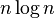average case :worst case :Quick sort  , who called it Quick ?

dunno . maybe it was the Quickest then ?  😀

kidding 😀 , Quick sort is one of the most efficient algorithms  it has n^2  as a worst case but it often reaches it’s worst case it’s also faster than those  nlogn algorithms in practice
because its inner loop can be efficiently implemented by most architectures ( array , linked list ..etc )

actually : Quick sort also  competes with merge sort  ,  that Quick sort could be implemented on Linked lists  and as well as that it takes a little Memory utilization needed usually ( Log(n) )  unlike merge sort wich needs  (n) extra memory when using arrays

let’s see how does Quick sort works :

Quick  sort Algorithm :

it is from (divide and conquer algorithms , like the  Merge sort )

1. Divide : using a pivot ( random number selected )
2. sort  all the numbers less than the Pivot on  before it
3. sort all the numbers greater than the pivot after it
4. then you have two arrays one all it’s element smaller than the pivot and the other larger than the pivot
5. repeat all those steps recursively on the two arrays

step  2  and  3  are done as following :

*we use two pointers , one points to the top of the array  ,and the other at the bottom  and in the middle lies the pivot  move the left pointer until you find an element greater than the pivot ( it should to to the next side )

* on the other side move the right pointer until it hit a number less than the pivot  ( it should go to the next side )

*replace the two numbers

*  repeat those steps until you reach the pivot then the left array will become less than the pivot , and the right is more than the pivot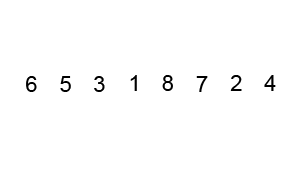check this video it elaborates will the using of two pointers :

you can check this video for further demonstration  and some code snippets :

_____________________________

# Heap Sort :

 best caseaverage caseworst case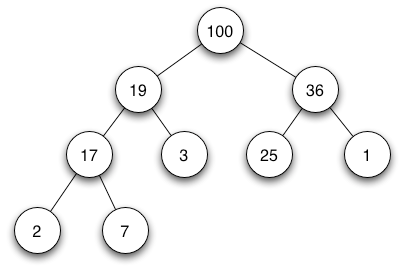this post is supposing that you have a pre-knowlege of what’s heap if you don’t , you can check this video  or this

Heap sort is an efficient  kind  of  Selection sort  ( yeah the Dumbest one ever ) 😀

How ? ?

in selection sort we used to search for the smallest number in the array  so it was a complex algorithm of complexity n^2

but in heaps you can easily search for elements inside it .  i needs number of iterations = height of the Tree = log(n)

so it Gives an overall complexity of  O(nlog(n))

### this is how it works

• Step 1: Build a heap
• Step 2: removeMin()

the minimum item in the heap will be always the Root of the Heap so we will always remove it and reconstruct the heap again and it will be super easy when you are dealing with a Heap class that have remove Function

### removing item from the heap

when you remove the root of the heap you and replace it with the last element in the heap

then you compare it with it’s two children , and replace the root with the minimum of it’s two children

then repeate those steps with the replaced item untill the root is smaller than it’s children

( if you don’t Get this , it’s recommended to revise the Heap removal )

the worst case here is when you compare the item to the Bottom element of the TREE , then you will do log(n) iterations

for removing n items you will have and overall complexity of O(nlog(n)) which is the complexity of the heap sort

comparing to the Selection sort (O(n^2)) it’s a Huge progress, although both based on the same logic

__________________________________________________________________

## till now we are Done 🙂

yet let’s do some conclusions 😀
from different searching algorithms above and in the last post we found that

in the average case :

our three heroes will be the : Merge sort , Quick Sort , Heap sort

and our Loosers will be : the Bubble sort and the Selection sort and the insertion sort

in case of a preordered array :

the true heroes will be : the bubble and the Intersion sort for having a O(n) complexity

and the looser will be : the dumb turtle 😀 the Insertion sort

Finally that most of us before using the Algorithms was using the Bubble sorting algorithm to sort Arrays

so it once again will be the Hero as the Most Famous Sorting Algorithm

______________________________________________________________

## AlgoRythmics :those people who do folk  algorithmic dances watch their youtube channel

those Guys are awesome 🙂

it’s the best practice now to watch their Dances and try to map them to what you’ve  learnt on different sorting algorithms## ________________________________

### and once again Credit Goes to :

• MIT opencourse ware :  introduction to algorithms course , with charles and Eric  🙂  i recommend to watch the Full playlist  or take a look on  the full course with the slides and exercises  the from the MIT opencourseware website  here  thank you MIT 🙂
• thanks to wikipedia for the Amazing animated Gif  images  🙂
• Google for images of  Rabbits and Bunnies  😀

# why people are in need of different sorting algorithms ?

there are a lot of metrics to compare sorting algorithms on  like :

• complexity ( usually it resembles the time taken in sorting ) in the best cases , worst cases & random cases as well
• memory usage < some sorting algorithms need to make a temporary locations to store data which needs more memory >
• adaptability  < there are some sorting algorithms that benefits from the presorted elements even they have high complexity  > it’s not reasonable to resort millions of entries when one element only is not sorted
let’s take a look how complexity could be a big deal :
if we have 3 sorting algorithms  n , nlogn , n^2

#### 10^16

if we used the 3 sorting algorithms above to sort 10^8 elements   :

if  the first algorithm of complexity n  took 27 hours

the second one of complexity nlogn  will take 8 times the time above 222 hours  ,

But the third algorithm  could take about  325114 YEARS  !!!!!!!!!before Going through different sorting algorithms , i wanted to share a Funny Video that demonstrates

3 different sorting algorithms 🙂 , it will ease explanation of them afterwards .

# Bubble sort  (  complexity O(n^2 ) ) :Bubble sort best case complexityaverage case complexityworst case complexityadvantages :  Tiny code size

it’s a simple sorting algorithm to write it’s code yet it’s  very complex algorithm , it could take days to sort few millions of elements

even  Obama Know this  😀

### it depends on 3  simple steps :

repeat those 3 steps until the array is sorted :

1. loop on the array
2. compare each to consecutive elements , and switch them if they are not sorted
3. go to step 1

watch this video , it explains  a lot  ( you can skip the other kind of sorting )

you can check it’s code

void bubbleSort (Array S, length n) {
boolean isSorted = true ;

while(!isSorted) { // repeat until it's sorted isSorted = true;
for(i = 0; i<n-1; i++) { // step one loop on the elements

if(S[i] > S[i+1]) // compare each two consecutive elements
{
int temp = S[i];
S[i] = S[i+1];
S[i+1] = temp ;

isSorted = false; // if all elements are sorted the flag wont change
}
}
}
n--;
} 

the best case here is when the array is already sorted or  consecutive elements are only unsorted  so it will be sorted by one while  loop only so it has complexity

the worst case  here will occur if the array is reversed  , you will need to loop in the while loop for n times so it’s complexity will be n^2

# insertion sort  (O(n^2)) :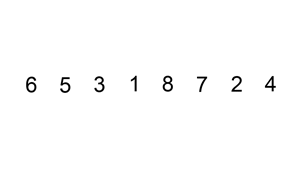Insertion sort best caseaverage caseworst caseso it’s a  basic algorithm like sorting a pile of cards ,from left to right  you pick a card and insert in into the right place then the preceding another card  and so on till the pile is sorted

take a look on this video

yet another way of stupid slow sorting  algorithm 😀   the only difference than before is that Obama forgot to mention it  kidding 😀

actually insertion sort is faster than bubble sort when dealing with presorted elements in the array  , how ??

imagine you are handling the element that already in sorted in it’s place ,

all that you need it to compare it to the largest element in the left sorted array to recognize it’s bigger than it and it’s in the right place

# Merge sort  ( O(nlog(n))  ):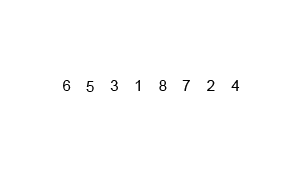Merge sort Best case  n log (n) average case  n log (n) worst case  n log (n) advantages : too fast compared to n^2 complexity

it’s considered as a fast search algorithm and least complexity , it  depends on a basic rule , is that sorting and merging small arrays is too much faster than sorting large array .

it uses 3 basic rules :

• arrays of one element only are already sorted
• divide any array into two halves and so on till you have  n arrays  each of one element
• merging each two consecutive arrays  in order //  so if we need to merge those two arrays in figure we will need to compare the first elements in each array then drop down the smaller into the new arraythen compare the next two selected elements  and so on till the whole array is merged

take a look on this video :

so why it has complexity of  nlog(n) ??? :

each level   consists of n elements  ,  merging depends on comparison of each to elements  so merging each  level will have complexity of  O(n)   and since we have  log(n) levels  , so merging will be repeated log(n) times
over all we will have complexity of  O(nlog(n)) .…to be continued with more Sorting algorithms  ( quick sort , selection sort , heap sort , BST sort  )  =)